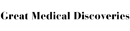# Number Theory - Research Article from Great Medical Discoveries

This encyclopedia article consists of approximately 4 pages of information about Number Theory.
 This section contains 898 words (approx. 3 pages at 300 words per page) View a FREE sampleNumber theory is a broad and diverse part of mathematics that developed from the study of the integers. In this article we describe two kinds of problems that have stimulated the development of number theory.

A very old problem in number theory is to determine the integer solutions to equations or systems of simultaneous equation. Equations to be solved in integers are called Diophantine equations after Diophantus of Alexandria. Diophantus probably lived about 250 a.d. He is known for his work Arithmetica in which he posed and solved various types of equations in integers. This part of number theory has strong connections to algebraic geometry and is sometimes referred to as Diophantine geometry. In some cases it is possible to completely determine all integer solutions to a particular Diophantine equation. For example, it is possible to describe all of the triples of integers (x, y, z...

(read more)

 This section contains 898 words (approx. 3 pages at 300 words per page) View a FREE sampleMore summaries and resources for teaching or studying Number Theory.
CopyrightsNumber Theory from Lucent. ©2002-2006 by Lucent Books, an imprint of The Gale Group. All rights reserved.